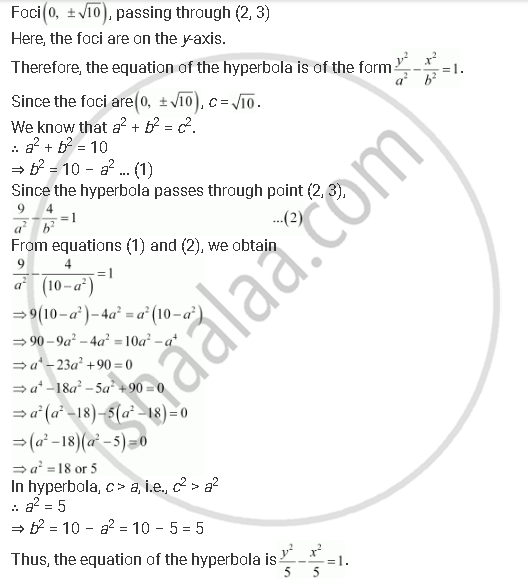CBSE (Arts) Class 11CBSE
Share
Notifications

View all notifications
Books Shortlist
Your shortlist is empty

# Find the Equation of the Hyperbola Satisfying the Give Conditions: Foci (0, +- Sqrt10), Passing Through (2, 3) - CBSE (Arts) Class 11 - Mathematics

Login
Create free account

Forgot password?
ConceptHyperbola Standard Equation of Hyperbola

#### Question

Find the equation of the hyperbola satisfying the give conditions: Foci (0, +- sqrt10), passing through (2, 3)

#### SolutionIs there an error in this question or solution?

#### APPEARS IN

Solution Find the Equation of the Hyperbola Satisfying the Give Conditions: Foci (0, +- Sqrt10), Passing Through (2, 3) Concept: Hyperbola - Standard Equation of Hyperbola.
S